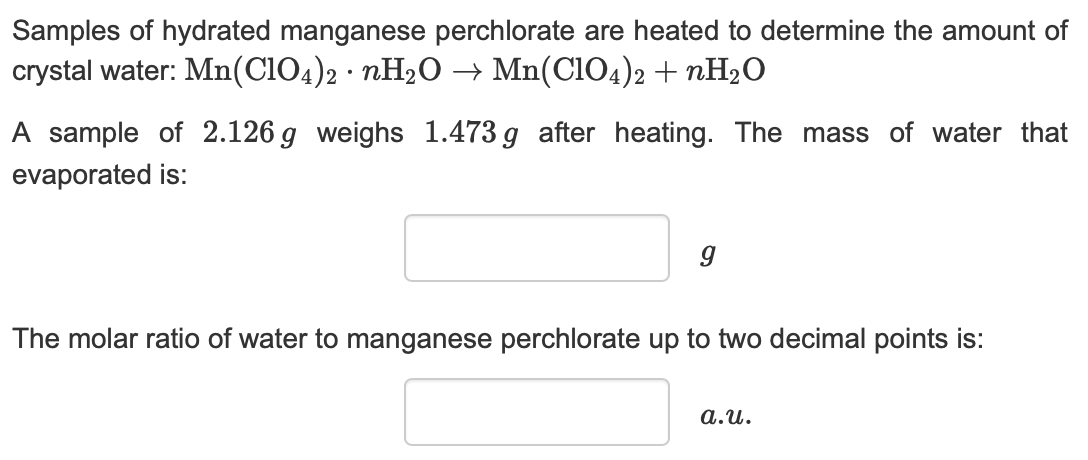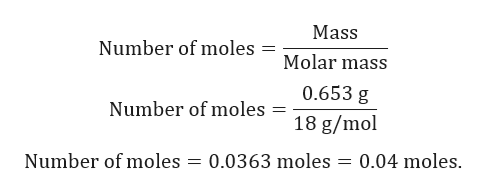Samples of hydrated manganese perchlorate are heated to determine the amount ofcrystal water: Mn(CIO4)2 nH20 -» Mn(CIO4)2 nH20A sample of 2.126 g weighs 1.473g after heating. The mass of water thatevaporated is:The molar ratio of water to manganese perchlorate up to two decimal points is:a.u

Questionhelp_outlineImage TranscriptioncloseSamples of hydrated manganese perchlorate are heated to determine the amount of crystal water: Mn(CIO4)2 nH20 -» Mn(CIO4)2 nH20 A sample of 2.126 g weighs 1.473g after heating. The mass of water that evaporated is: The molar ratio of water to manganese perchlorate up to two decimal points is: a.u fullscreen
Step 1

Given:

Mass of hydrate before heating (Mn(ClO4)2.nH2O) = 2.126 g

Mass of hydrate after heating Mn(ClO4)2 = 1.473 g.

Chemical reaction is given by:

Step 2

Calculation for mass of water:

Mass of water = Mass of hydrate before heating (Mn(ClO4)2.nH2O - Mass of hydrate after heating Mn(ClO4)2

Mass of water = 2.126 g – 1.473 g = 0.653 g.

Step 3

Calculation for number o...help_outlineImage TranscriptioncloseMass Number of moles = Molar mass 0.653 g Number of moles 18 g/mol 0.04 moles Number of moles 0.0363 moles fullscreen

Want to see the full answer?

See Solution

Want to see this answer and more?

Our solutions are written by experts, many with advanced degrees, and available 24/7

See Solution
Tagged in

General Chemistry